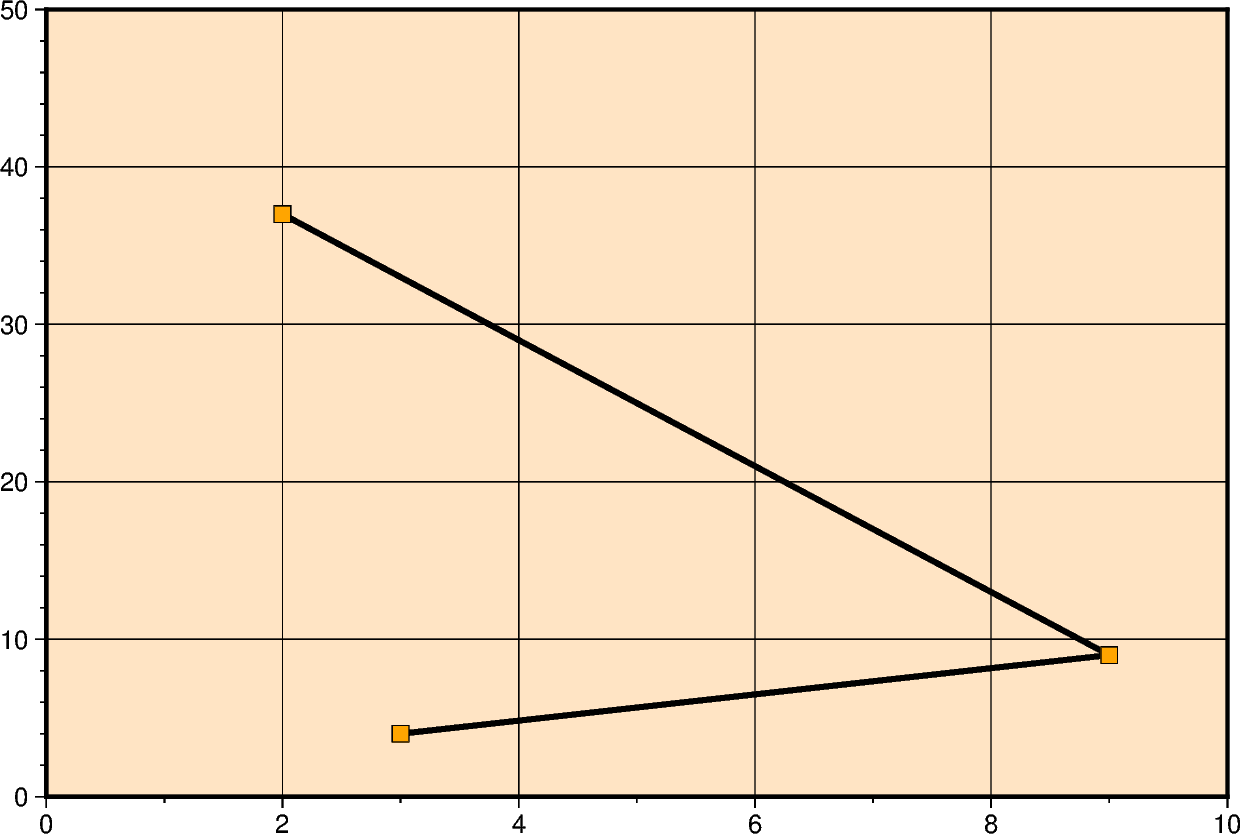# Cartesian linear¶

Xwidth/[height]: Give the width of the figure and the optional height.Out:

```<IPython.core.display.Image object>
```

```import pygmt

fig = pygmt.Figure()
fig.plot(
# The x and y parameters determine the coordinates of lines
x=[3, 9, 2],
y=[4, 9, 37],
pen="3p,red",
# ``region`` sets the x and y ranges or the Cartesian figure.
region=[0, 10, 0, 50],
projection="X15c/10c",
# ``WSne`` is passed to ``frame`` to put axis labels only on the left and bottom axes.
frame=["af", "WSne"],
)
fig.show()
```

Total running time of the script: ( 0 minutes 1.048 seconds)

Gallery generated by Sphinx-Gallery